# Solving Linear Equations

A collection of resources to use to demonstrate and practise solving equations.

Student Exercises
I find Owen Elton’s worksheet, Balancing Equations on TES Resources very useful when introducing equations, the diagrams emphasise that we must do the same to both sides.
(See Diagrams in Mathematics, for more on the use of diagrams to help understanding).

As an alternative to the balance approach, consider this doing / undoing approach, described here by Don Steward, this is an approach I use for finding inverse functions. The exercises Don refers to are here.

One of the Standards Unit resources, A2 Creating and solving equations (in Mostly Algebra) uses this approach, students create an equation and  then undo it; this is a great exercise for demonstrating notation. Another resource with this approach comes from the Mathematics Assessment Project (the design and development was led by the MARS Shell Center team at the University of Nottingham) Building and Solving Linear Equations lesson

For a superb collection of ideas and student exercises for solving linear equations see all Don Steward’s posts tagged linear equations. Many of these outstanding resources use a very visual approach with very clear diagrams to help students’ understanding.

There are numerous questions on linear equations on Diagnostic Questions.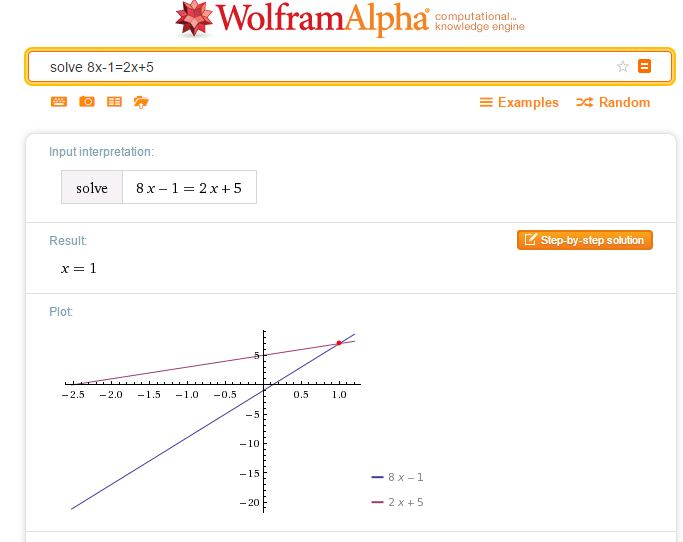A simple way to check a solution to an equation of any type is to simply enter your equation as a WolframAlpha query. Note that WolframAlpha includes a graphical illustration; it is so important for students to understand how equations may be solved graphically; I always illustrate graphical solutions when we are working with Algebra to help students make these links. Desmos of course, is ideal here.The following three resources work well for demonstrating the balance method of solving linear equations.

Duncan Keith’s Linear Equation Calculator is available on STEM Learning.Choose the type of equation you require then the sequence of operations required to solve the equation.
Select Do it after each operation, for example -32 Do it were the keys selected to start the above problem.

The slideshow below shows how to use the calculator to solve equations where the unknown is on both sides.

Mathisfun

Mathisfun has this very clear and easy to use interactive illustrating the solution of linear equations.

# Diagrams in Mathematics

Seeing this problem on Brilliant recently reminded me how useful diagrams can be in the study of Algebra. I solved the problem using Algebra (with a little colour for clarity!) as follows:

Sybilla Beckmann’s paper, Solving Algebra and Other Story Problems with Simple Diagrams: a Method Demonstrated in Grade 4–6 Texts Used in Singapore is an interesting read on the subject of the use of diagrams.

A clear diagram can be so helpful in understanding a problem, look what one of my Year 7 students did when asked for the nth term of a sequence having been given a diagram:For more Algebra with diagrams try Mobile Puzzles a collection of problems varying in difficulty for simple for young students to rather more complex.

Algebra Tiles are such a good way to demonstrate algebraic manipulation, see the online demonstration on MathsBits.com.

Write x2+6x+11 in the form (x+a)2+bComplete the square – use algebra tiles

And From Fawn Nguyen comes the brilliant Visual patterns, note the menu; the Gallery includes blog posts from teachers and students who’ve used visual patterns in their classrooms.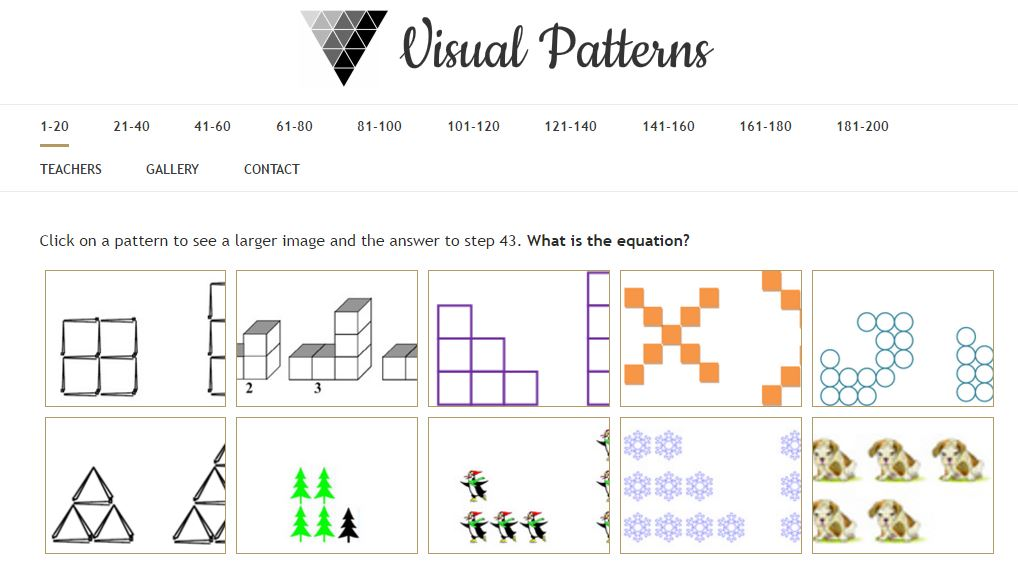…and for some lovely visualisations have a look at this post.

# Algebra – expand three brackets

Following a recent test for Year 10 (UK age 14-15) I wanted to use colour to show clearly a way to make sure all terms have been included when three brackets have to be expanded (new to the UK GCSE qualification). (Colour can be so useful in Mathematics – something I have written on before).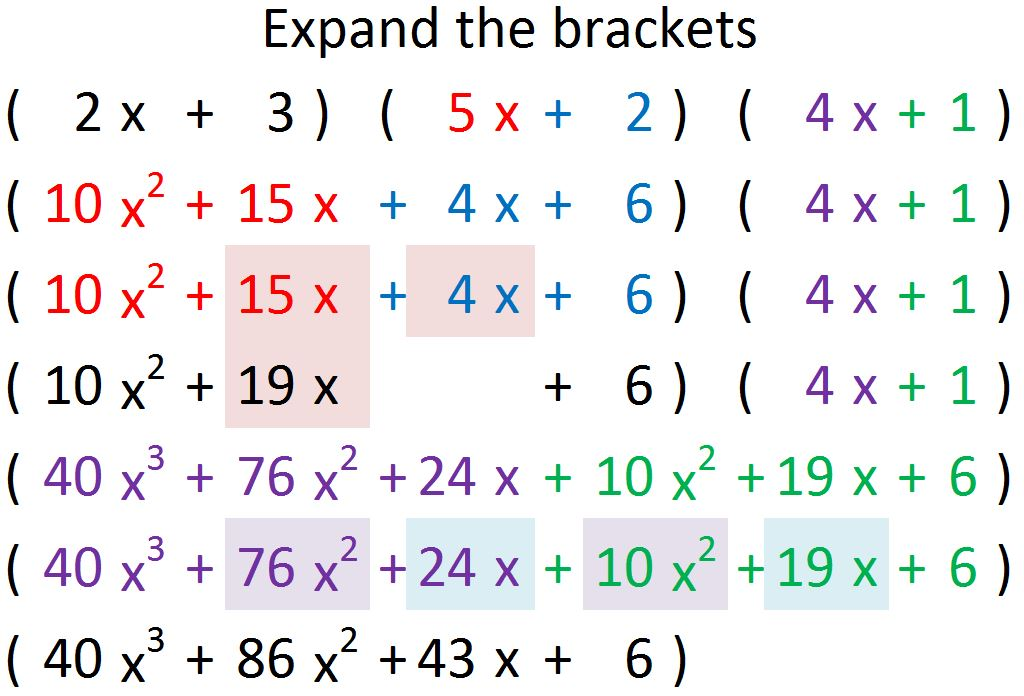I have created this example using Excel. This is just a simple example with positive coefficients only; I wanted to start by being clear on making sure that all combinations are included. There are various FOIL and quick methods available if you search but my own preference is a more systematic approach. An advantage of using Excel is the ability to trace precedents (Formulas menu).The numbers could be changed and of course any examples could be made up and checked on WolframAlpha.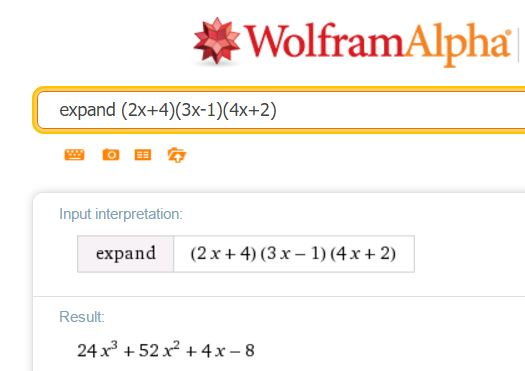I have also collated some Algebra resources (many topics) – examples and exercises on Mathematics for Students. Note the very clear workbooks from Plymouth University

# FunctionsA previous post on Functions features resources aimed at older students; I thought it would be useful to look at resources for teaching younger students.

For an excellent introduction the PhET Simulation, Function Builder provides a very visually appealing resource. Using the pattern option even very young students could explore important concepts. This is very intuitive to use, the slides in the following slideshow demonstrate examples of its use. Note that teachers can register with PhET and gain access to supporting documentation.

Desmos can be used very simply to illustrate function notation and note the use of Desmos as a calculator to evaluate the value of a function for a given input.

As always CIMT is worth a search; we find:

In the interactive materials for year 7: Unit 16 Section 2: Function Machines and there is also a section in the associated text (16.2). All CIMT resources are free to access, a small number of documents such as text answers are password protected, you can obtain the CIMT password. These exercises could be used as an introduction and students also shown function notation. They could write the functions described by the function machines using f(x) notation.

On Transum, try this online exercise on function notation, inverse functions and composite functions.

On Maths Genie, under Grade 7, we have Inverse and Composite Functions. You will find some revision examples and also exam questions with solutions.

From Don Steward, try these lovely questions on Compound Functions.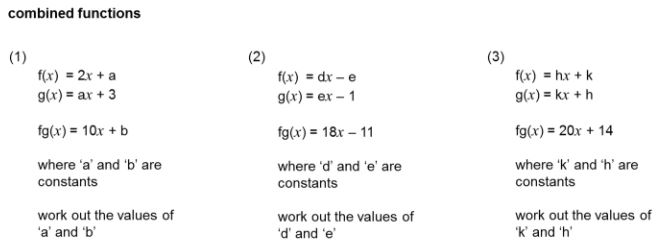On TES, this Crossnumber from cbarthur is an ideal resource for becoming familiar with function notation. Also on TES from Owen134866 we have a set PowerPoint resources including an introduction to f(x) notation for GCSE students.

The AQA Bridging the Gap resources includes a resource on introducing function notation; these resources are ideal for students who have completed the previous KS3 programmes of study but will be studying the new GCSE courses.

From OCR, it strikes me that the Check in tests will be very useful in KS3, consider the language of functions for example, the first questions could be used for students as young as Year 7 (age 11-12).

.

On Exam Solutions you will find an introduction to f(x) notation.

# GCSE New Content – Iterative Methods for Numerical Solution of Equations

(Updated May 2017 with Transum resources in the Further Resources section, March 2017 with Edexcel’s New Content Resources and further TES resources and November 2016 – Diagnostic Questions added to Further Resources /Questions section.)

Looking at the new content for UK GCSE Mathematics a completely new entry on the specification is “find approximate solutions to equations numerically using iteration”.

For some more information on this AQA have some very useful resources, including their Bridging the Gap resources which look very useful for students who have studied the 2007 Key Stage 3 Programme of Study and will be studying a new  Mathematics GCSE specification. The resources include examples on iterative methods for solving equations numerically.

Students can be reminded to use the ANS key on their calculators; it seems to me that this will be a good opportunity to show students how useful Excel can be for such techniques and will enable teachers to quickly generate results with different starting values.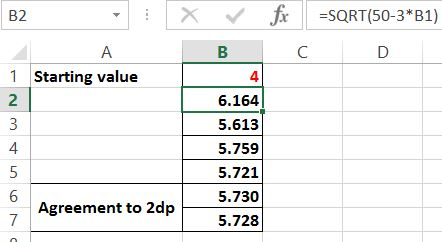From an AQA specimen paper, we see how this may be examined: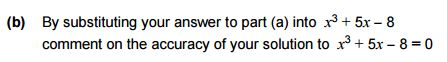In case you are wondering about that flowchart, Newton-Raphson is the method being used.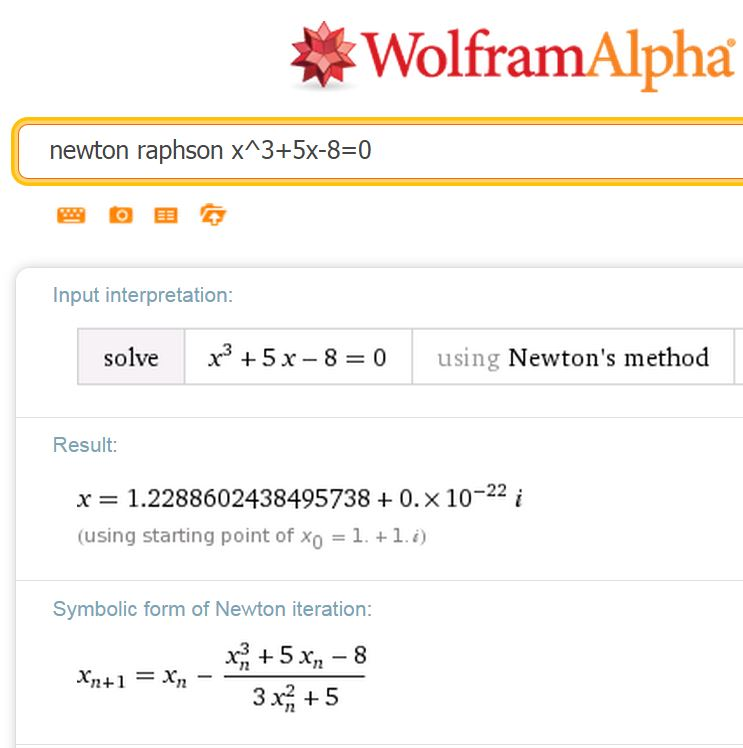a little algebra and we see what AQA are up to in their flowchart.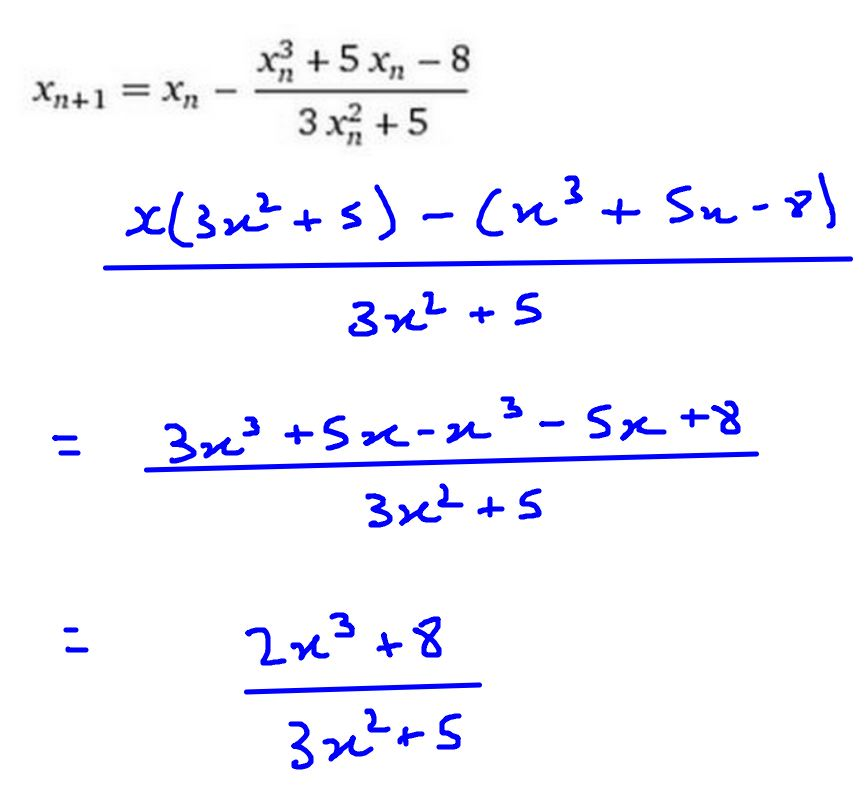I do love my graphics tablet!
(See Writing Maths Online)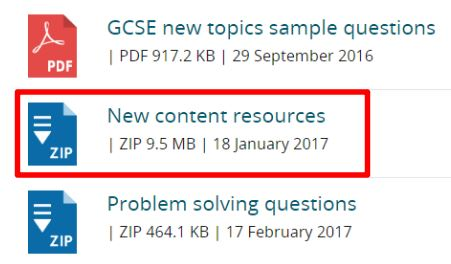Looking at Edexcel’s Content Support you will find very helpful resources for teaching new content. For new content, information, examples and exercises (with answers) are given. This includes Iteration. Scroll down the list for a zipped file.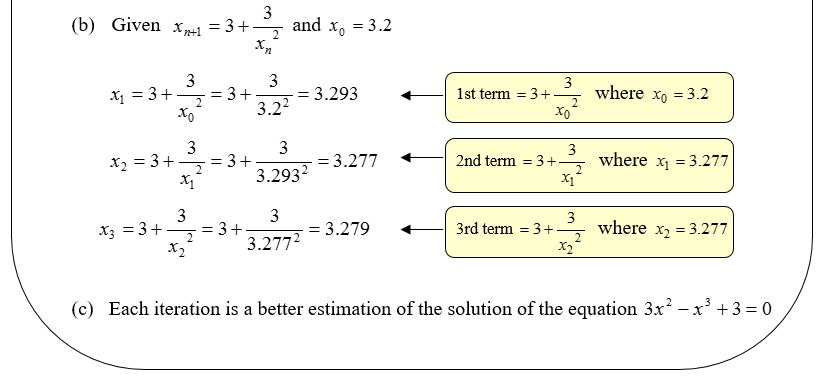Further Resources / Questions

In this post I have included fully worked examples and related graphs; this includes an example (note the pdf file) where an equation has been solved using trial and error and then rather more efficiently using an iterative technique.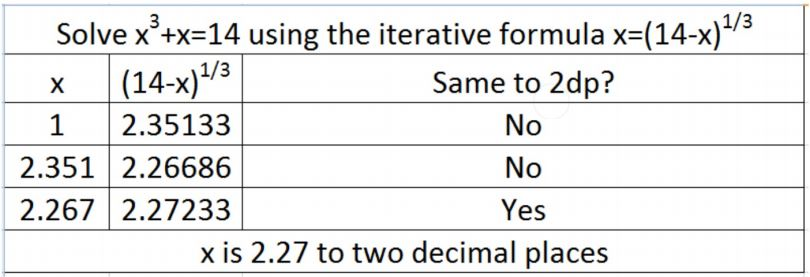Diagnostic QuestionsOn the brillant Diagnostic Questions site you will find excellent coverage of topics new to the GCSE specification. You can also search all questions for a topic of your choice, for example a search on iteration will lead you to the whole collection of Trial and Improvement and Iterative Methods questions. (You will need to be logged in  to the site to follow the link. Create an account if you have not already done so as this site with thousands of high quality diagnostic questions and additional analytical features is free. If you scroll down the page you’ll see that Diagnostic Questions are giving “you, the teacher in the classroom, a promise that Diagnostic Questions will always remain free.”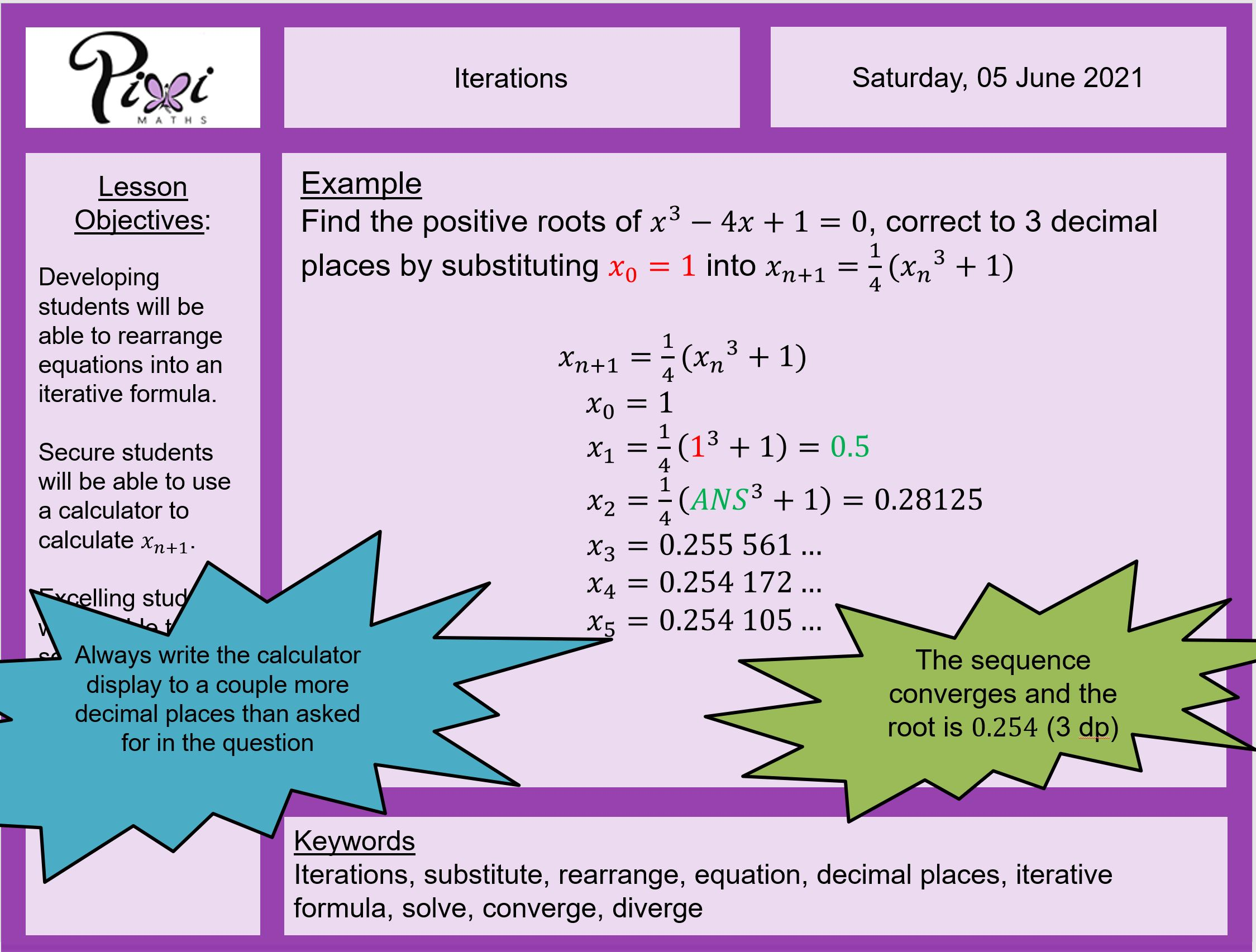From piximaths – see Iterations.

Also on TES Resources dannytheref has a very clear PowerPoint and accompanying worksheet on how to answer iteration questions.This search on TES returns some further free GCSE Iteration resources including a very clear introduction, Iteration and Square Roots from Owen134866 and a Tarsia puzzle from Jill Hillitt which provides several examples.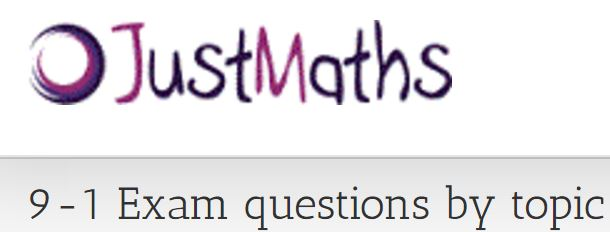On Just Maths we have so many wonderful resources including 9-1 questions by topic, looking at the Higher Tier questions, note that under Algebra (scroll down) Iteration – questions and solutions are available. Questions from Edexcel, AQA and OCR are included.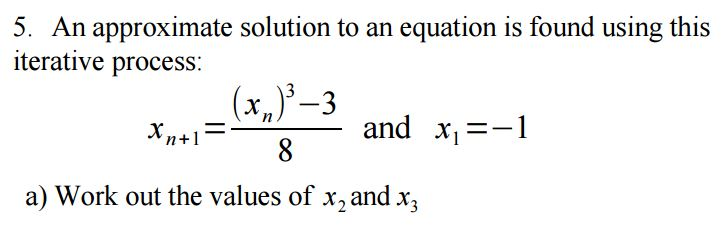Maths Genie

The Maths Genie website includes numerous questions and solutions; on this page, scroll down to the last section for Higher and note that under Algebra we have for Solving Equations Using Iteration, revision examples, examination questions and solutions. Looking at the examination questions, the first three questions use Trial and improvement, questions 4 to 7 are on iterative techniques.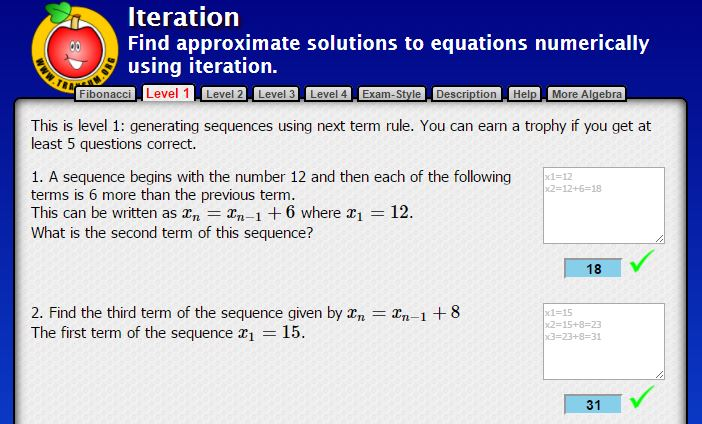For more questions Transum Mathematics offers the exercises shown below on Iteration:
Level 1 – Generating sequences using next term rule.
Level 2 – Rearranging equations.
Level 3 – Using flowcharts to define iterations.
Level 4 – Solving Equations to 1dp.

Level 1 will be useful for KS3, students can find the notation very unfamiliar for sequences.